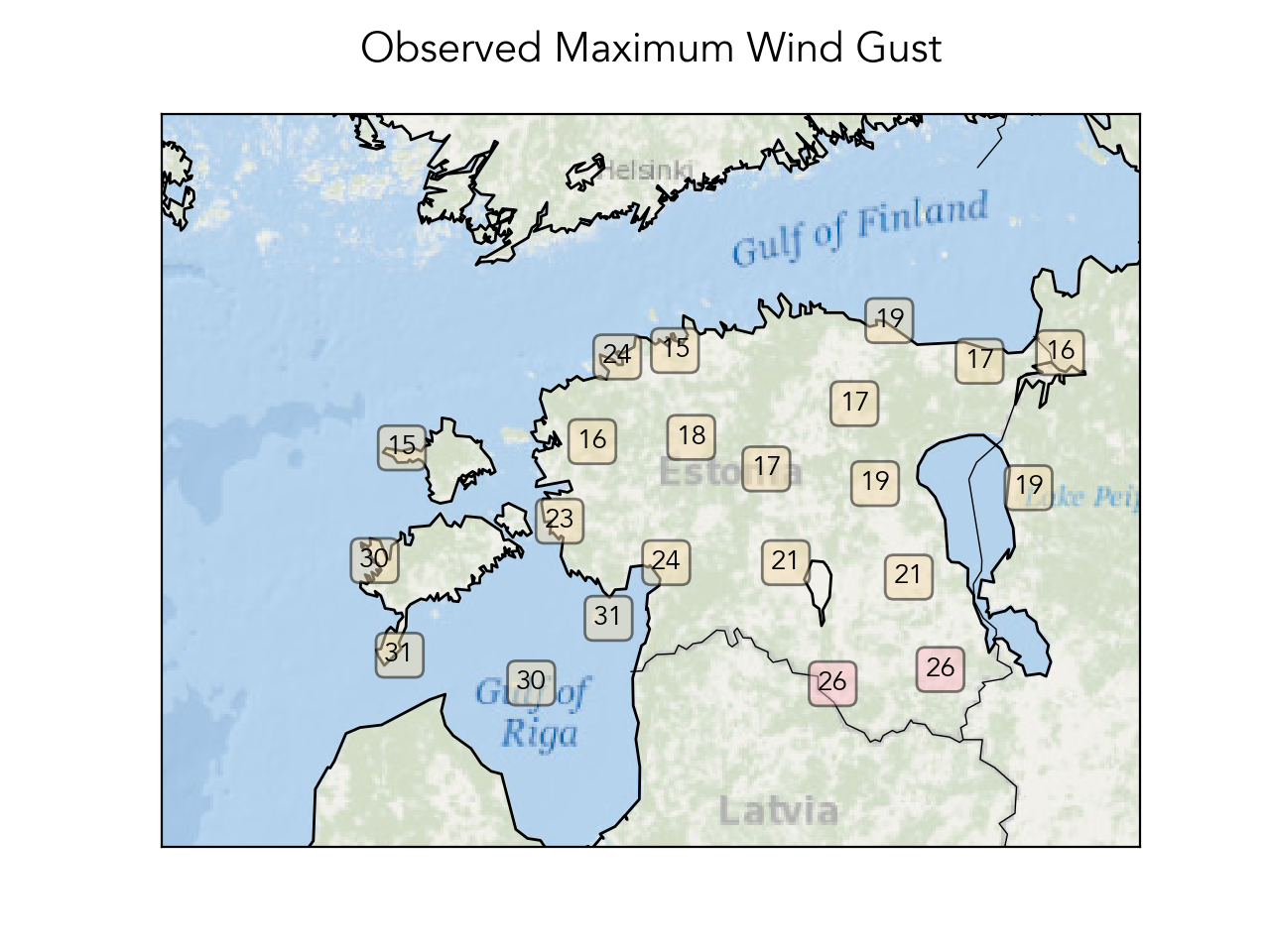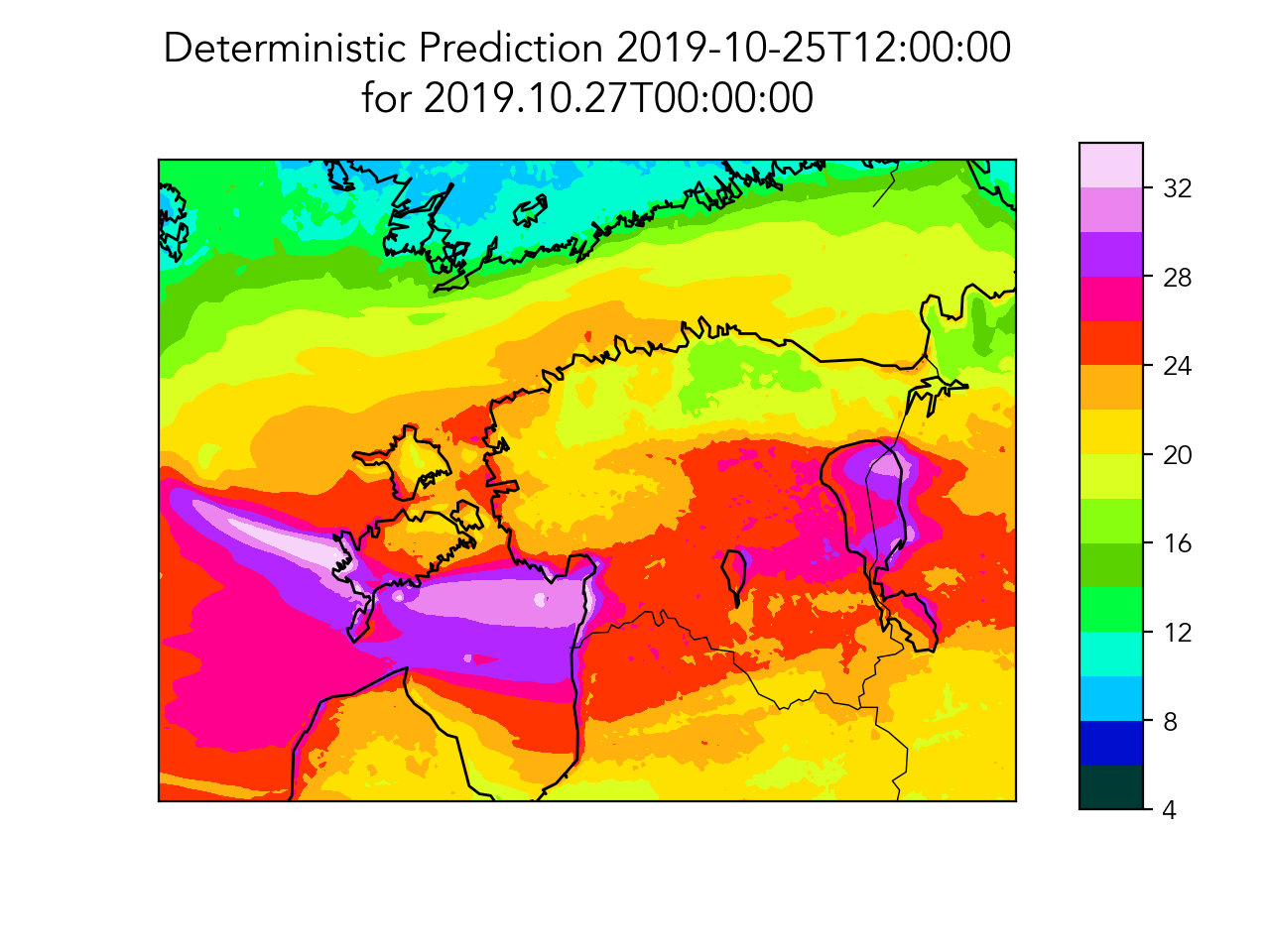# Using Ensemble Forecasts for Extreme Weather Early Warning Systems¶

In this notebook, we are showing how to use MetNO Harmonie Ensemble Forecast. Example is based on a storm that happened on 27th of October 2019, in Estonia and it was poorly forecasted and warnings that were put out, were not strong enough.

If you have questions or comments, join the Planet OS Slack community to chat with our development team. For general information on usage of IPython/Jupyter and Matplotlib, please refer to their corresponding documentation. https://ipython.org/ and http://matplotlib.org/

This Notebook is running on Python3.

In :
%matplotlib notebook
from API_client.python.datahub import datahub_main
from API_client.python.lib.dataset import dataset
import xarray as xr
from mpl_toolkits.basemap import Basemap
import matplotlib.pyplot as plt
import numpy as np
import datetime
import glob
import os
import matplotlib
import pandas as pd
matplotlib.rcParams['font.family'] = 'Avenir Lt Std'
print('matplotlib version: ' + matplotlib.__version__)

matplotlib version: 3.1.2


We are downloading the data from met no thredds as it's not available on Planet OS Datahub yet, but will be available soon.

Note that it might take some time as the data amount is quite big and it also depends on your network speed.

In :
timerange = slice('2019-10-27T00:00:00', '2019-10-28T00:00:00', None)
start = datetime.datetime(2019,10,25,12)
end = datetime.datetime(2019,10,27,6)
while start <= end:
if not os.path.exists(os.getcwd() + "/wgust_{day}_{hour:02d}.nc".format(day=start.day, hour=start.hour)):
wds = xr.open_dataset('http://thredds.met.no/thredds/dodsC/meps25epsarchive/2019/10/{day}/meps_extracted_2_5km_201910{day}T{hour:02d}Z.nc'.format(day=start.day, hour=start.hour))
wdata = wds.wind_speed_of_gust.sel(time=timerange).compute()
wdata.to_netcdf("wgust_{day}_{hour:02d}.nc".format(day=start.day, hour=start.hour))
else:
print('Data for ' + str(start) + ' forecast run already exists')
start += datetime.timedelta(hours=6)

Data for 2019-10-25 12:00:00 forecast run already exists
Data for 2019-10-25 18:00:00 forecast run already exists
Data for 2019-10-26 00:00:00 forecast run already exists
Data for 2019-10-26 06:00:00 forecast run already exists
Data for 2019-10-26 12:00:00 forecast run already exists
Data for 2019-10-26 18:00:00 forecast run already exists
Data for 2019-10-27 00:00:00 forecast run already exists
Data for 2019-10-27 06:00:00 forecast run already exists


There might be a case where Met NO thredds server is down. If so, try to download data from S3.

In :
# import boto3
# import botocore
# BUCKET_NAME = 'data.planetos.com'
# s3 = boto3.resource('s3')

# timerange = slice('2019-10-27T00:00:00', '2019-10-28T00:00:00', None)
# start = datetime.datetime(2019,10,25,12)
# end = datetime.datetime(2019,10,27,6)
# while start <= end:
#     print('Data for ' + str(start) + ' forecast run already exists')
#     if not os.path.exists("wgust_{day}_{hour:02d}.nc".format(day=start.day, hour=start.hour)):
#         KEY = 'import_sources/met_no_estonia_storm/wgust_{day}_{hour:02d}.nc'.format(day=start.day, hour=start.hour)
#         try:
#         except botocore.exceptions.ClientError as e:
#             if e.response['Error']['Code'] == "404":
#                 print("The object does not exist.")
#             else:
#                 raise
#     start += datetime.timedelta(hours=6)


Here we initialize dataset. Also, please put your datahub API key into a file called APIKEY and place it to the notebook folder or assign your API key directly to the variable API_key!

In :
apikey = open('APIKEY').readlines().strip()
dh = datahub_main(apikey)
ds = dataset('noaa_rbsn_timeseries',dh)


Now, we get stations and clean the list to have only stations with spatial extent. We also define a function for finding out if station is in our desired area or not. The area defined here is Estonia. Then we filter out Estonian stations.

In :
stations = ds.stations()
stations_clean = {i:stations['station'][i] for i in stations['station'] if 'SpatialExtent' in stations['station'][i]}

def proxim(in_coords):
lon, lat = in_coords

lonstart = 20.5
latstart = 57
lonend=28.4
latend = 59.7
if lon >= lonstart and lon <= lonend and lat >= latstart and lat <= latend:
return True
else:
return False
sel_stations = [i for i in stations_clean.keys() if proxim(stations_clean[i]['SpatialExtent']['coordinates'])]


We like to use Basemap to plot data on it. Here we define the area. You can find more information and documentation about Basemap here.

In :
m = Basemap(resolution = 'i', projection = 'lcc', \
llcrnrlon=20, llcrnrlat=57, urcrnrlon=29, urcrnrlat=60.5, \
epsg = 3395)


Getting gust variables with data

In :
gust_hours = [0,0.5,1,2,3,4,5,6,12,18]
variables = ["highest_wind_gust_{}_hour".format(i) for i in gust_hours]
variables.append('station_name')
st_pandas = ds.get_station_data_as_pandas(station_list=sel_stations, variables=variables,start='2019-10-25T00:00:00')

In :
st_pandas_2 = {}
gust_vars = ["highest_wind_gust_{}_hour".format(i) for i in gust_hours]
for kk in st_pandas.keys():
tempp = st_pandas[kk].dropna(axis=1, how='all')
if len([i for i in gust_vars if i in tempp.columns]) > 0:
st_pandas_2[kk] = tempp


Making map with highest observed wind gusts on 27th of October. Võru and Valga is made red, because most of the damage happened there. It is inland and 26 m/s is a long time record for those places (there hasn't been such high winds over 50 years). Roofs were flying and many trees fell down. Electricity was lost in more than 60,000 housholds, the vast majority of this coarsely populated area. And for many, the ensuing power outages lasted for more than three days.

In :
def xy(mp, coords):
x,y = coords['SpatialExtent']['coordinates']
return mp(x,y)

fig = plt.figure()
props = dict(boxstyle='round', facecolor='wheat', alpha=0.5)
obs_dict = {}
for i in st_pandas_2:
pdi = st_pandas_2[i]
pdtest = pdi[(pdi.index >= '2019-10-27T00:00:00') & (pdi.index < '2019-10-28T00:00:00')]
x,y = xy(m, stations_clean[i])
max_wind = 0
for gv in gust_vars:
if gv in pdtest:
max_wind = max(max_wind, pdtest[gv].max())
if np.unique(pdi['station_name']) == 'VORU' or np.unique(pdi['station_name']) == 'VALGA':
props = dict(boxstyle='round', facecolor='pink', alpha=0.5)
else:
props = dict(boxstyle='round', facecolor='wheat', alpha=0.5)

plt.text(x,y, "{0:0d}".format(int(max_wind)), bbox=props)
obs_dict[i] = max_wind

m.arcgisimage(service = "Ocean_Basemap")
m.drawcoastlines()
m.drawcountries()
ttl = plt.title('Observed Maximum Wind Gust',fontsize=15)
ttl.set_position([.5, 1.05])
plt.show()
#plt.savefig('obs.png',dpi=300,bbox_inches='tight')These two functions help to format time for images.

In :
def tformat(inn,title=None):
if title == None:
return datetime.datetime.utcfromtimestamp(inn.data.astype(int)/1e9).strftime("%Y.%m.%dT%H:%M:%S")#"%d.%m.%YT%H")
else:
return datetime.datetime.utcfromtimestamp(inn.data.astype(int)/1e9).strftime("%Y%m%dT%H")
def date_from_wgust_name(wgust_name):
splitted = wgust_name[6:11].split('_')
dat = '2019-10-' + str(splitted) + 'T' + str(splitted) + ':00:00'
return dat


Now we look into deterministic prediction made on 25th of October for 27 of October. Through the map below, it is evident that there will be strong winds on the western coast and surrounding islands, as well as in the central and southern parts of the country. In fact, it was found that both the distribution of strong wind areas and maximum values displayed by the forecast were similar to real-time observations.

In :
%%time
f='wgust_25_12.nc'
ftemp = xr.open_dataset(f)
a = ftemp.wind_speed_of_gust.isel(ensemble_member=0, height3=0).max(axis=0)
fig = plt.figure()
X,Y = m(ftemp.longitude.data, ftemp.latitude.data)
levels = range(4,36,2)
ppp0 = m.contourf(X, Y, a, cmap=plt.cm.gist_ncar, levels=levels)
m.drawcoastlines()
m.drawcountries()
ttl = plt.title("Deterministic Prediction {}".format(date_from_wgust_name(f)) + '\nfor ' + tformat(ftemp.time),fontsize=15)
ttl.set_position([.5, 1.05])
cbar_ax = fig.add_axes([0.85, 0.15, 0.05, 0.7])
fig.colorbar(ppp0, cax=cbar_ax)
plt.show()
#plt.savefig(f[:-3] + 'kontrollprogn.png',dpi=300,bbox_inches='tight')CPU times: user 519 ms, sys: 149 ms, total: 668 ms
Wall time: 1.21 s


In the next graphs below, we created supplementary forecasts that were issued every six hours and for the exact same time period (the day of October 27th, 2019). It should also be noted that as the forecast lead times decrease in duration, they typically become more accurate.

The first trends we can observe from these forecasts compared to the previous longer duration forecasts are weaker wind speeds both over the seas and inland. However, all forecasts do show maximum wind speeds over 20 m/s in the South-East part of the country. Friday evening and Saturday morning forecasts may easily leave the impression that the storm is weakening. So how should one interpret a situation like this, where subsequent forecasts change so significantly?

With this considered, we will now take a look at the ensemble forecast, rather than just the main deterministic forecasts.

In :
wgust_files = sorted(glob.glob("wgust_[0-9][0-9]_*nc"))
print (wgust_files)
levels=range(4,36,2)
fig=plt.figure(figsize=(8,12))
columns = 2
rows = 4
ax = []
for i,file in enumerate(wgust_files):
ftemp =